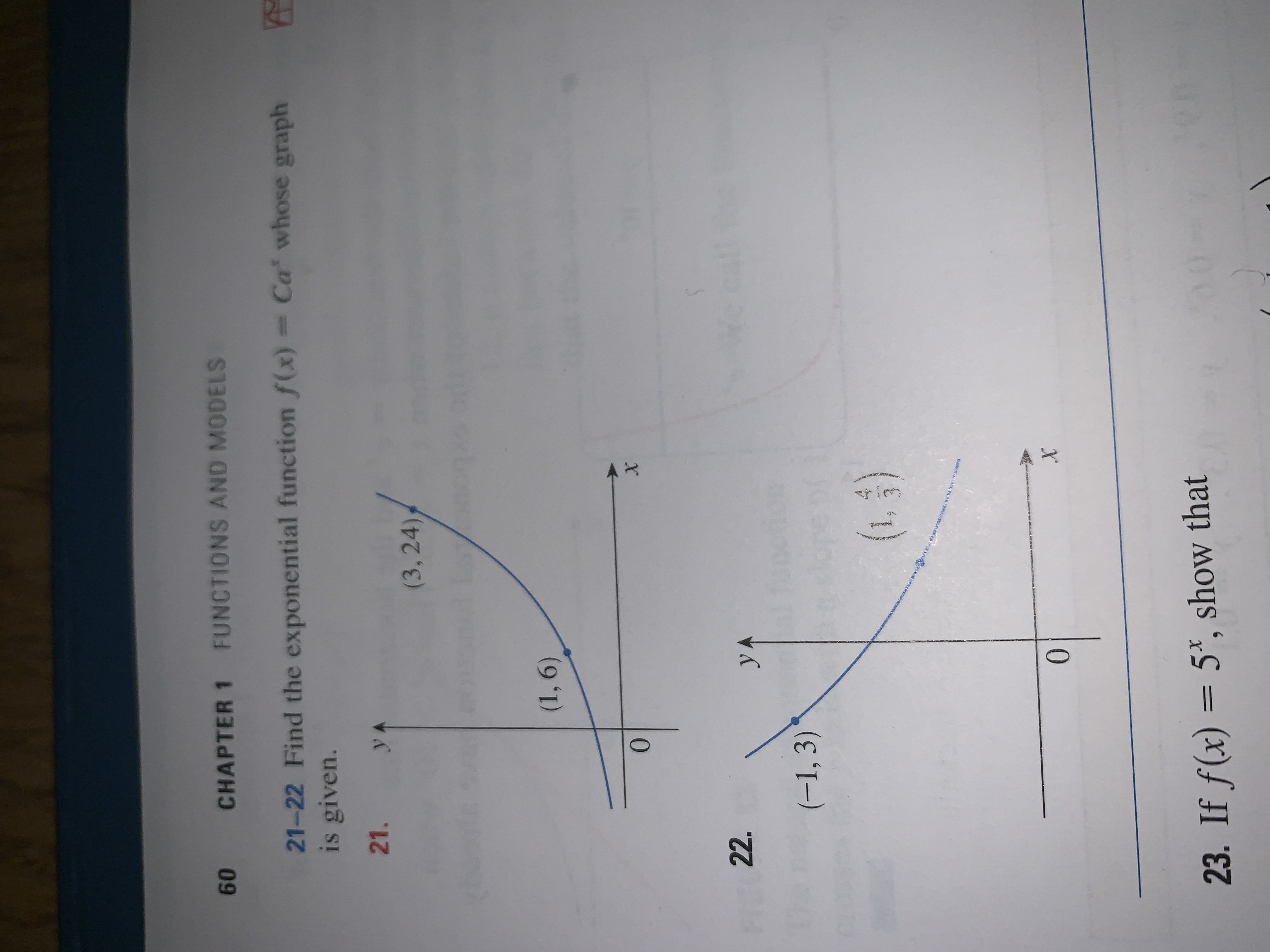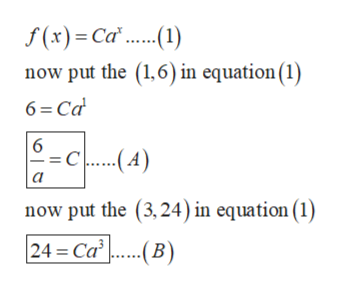# CHAPTER 1 FUNCTIONS AND MODELS6021-22 Find the exponential function f(x) = Ca whose graphis given.21.yA(3, 24);(1,6)0XFI 22.yAonctien(-1, 3)(.3)ENVnR BX023. If f(x) = 5*, show that

Question
3 views

How do I do these 2 questions? Math&151help_outlineImage TranscriptioncloseCHAPTER 1 FUNCTIONS AND MODELS 60 21-22 Find the exponential function f(x) = Ca whose graph is given. 21. yA (3, 24); (1,6) 0 X FI 22. yA onctien (-1, 3) (.3) ENVnR B X 0 23. If f(x) = 5*, show that fullscreen
check_circle

star
star
star
star
star
1 Rating
Step 1

Hello. Since you have posted multiple questions, but as per guidelines we can solve only one question so, we will solve the first question for you. If you want any other specific question to be solved then please resubmit only that question.

Now, coming to the question:

According to the given question, the exponential function of the graph is given below:

Step 2

The points on the graph will satisfy the equation of the graph.

Points on the graph are:

Step 3

So, put the points in th...help_outlineImage Transcriptionclosef(x) Ca.) now put the (1,6) in equation (1) 6 Ca 6 -C....-A) now put the (3,24) in equation (1) 24= Ca..B fullscreen

### Want to see the full answer?

See Solution

#### Want to see this answer and more?

Solutions are written by subject experts who are available 24/7. Questions are typically answered within 1 hour.*

See Solution
*Response times may vary by subject and question.
Tagged in

### Other